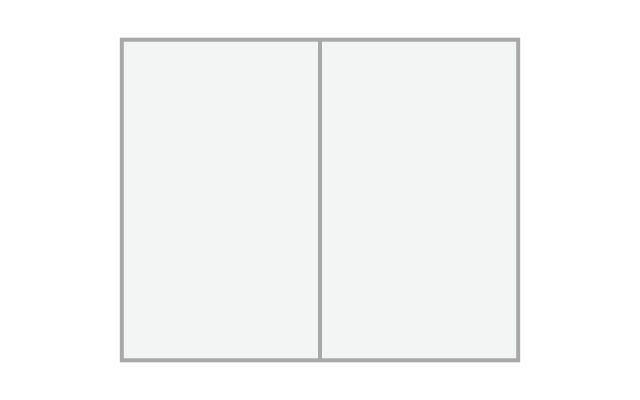# Ratios & Grids## golden ratio

a geometric proportion that has been theorized to be the most aesthetically pleasing to the eye (also known as golden section, golden mean, golden number, divine proportion, and phi)## golden rectangle

the “perfect” rectangle that uses the golden ratio; it is created using these steps:

• draw a perfect square and divide it in half
• draw a diagonal line that connects opposite corners of one half
• rotate the diagonal until it aligns with the side of the square
• extend the square to the end of the flattened diagonal## golden rectangle visual interest points

points are generated by drawing diagonals to the corners and putting focus on the halfway point to the center - this is sometimes referred to as the "eyes of the rectangle"## golden spiral

circular arcs are drawn connecting the opposite corners of intersections created by golden rectangles## root 2 rectangle

this rectangle can be infinitely subdivided into the same proportion; it is created using these steps:

• draw a perfect square
• draw a diagonal line that connects opposite corners
• rotate the diagonal until it aligns with the side of the square
• extend the square to the end of the flattened diagonal## double square

this rectangle is simply made of two perfect squares:

• draw a perfect square
• duplicate the square
• unite the two squares## fibonacci series

a series of numbers in which each number is the sum of the two preceding numbers## fibonacci spiral

circular arcs are drawn connecting the opposite corners of squares whose lengths are a sequence of fibonacci numbers## rule of thirds

dividing a composition into thirds (nine equal parts) and aligning important compositional elements to the imaginary lines or their intersections

## Grid Anatomy

Grids are used as guidelines for aligning elements. A page grid usually consists of the following:## margin

a distance from the edges (negative space) that defines the live area## modules

individual intervals of space, typically within the margins## gutter

the space between the modules## column

vertical module groups that create horizontal divisions## row

horizontal module groups that create vertical divisions## flow lines

horizontal lines created across the space between the margins## spatial zones

groups of adjacent modules that define a section## marker

a placement indicator for repeating elements, such as page numbers, running heads or footers

## Grid Types

Below is an introduction to different grid types. Grids can be simple or made very complex using a variety of shapes and sizes. Grids can also explore different angles as well as 3-dimensional space.## block grid

the simplest grid; elements run across the page to the margins - also called a manuscript grid## column grid

dividing a page into equal or unequal columns## modular grid

dividing a page horizontally and vertically into equally defined spaces## hierarchical grid

this is a custom grid used to intuitively place elements based on their position and dominancean elliptical division of the page - often used in conjunction with horizontal and/or vertical modules## triangular grid

dividing a page using triangles## classic proportion grid

above is another example of how a book's text block might be designed using divisions of the page to create a grid system